# Formula-driven graphics

There are several excellent graphics packages provided for R. The `ggformula` package currently builds on one of them, `ggplot2`, but provides a very different user interface for creating plots. The interface is based on formulas (much like the `lattice` interface) and the use of the chaining operator (`%>%`) to build more complex graphics from simpler components.

Experts, of course, will want to use the underlying `ggplot2` package directly in order to maximize expressiveness and minimize the “bureaucracy” induced by intervening layers of software. The `ggformula` graphics are suited for

• beginners, who don’t require the full range of `ggplot2` capabilities, but want to get started quickly,

• those familiar with `lattice` graphics, but wanting to be able to easily create multilayered plots,

• those who prefer a formula interface, perhaps because it is familiar from use with function like `lm()` or from use of the `mosaic` package for numerical summaries.

## The basic formula template

The basic template for creating a plot with `ggformula` is

``gf_plottype(formula, data = mydata)``

where

• `plottype` describes the type of plot (layer) desired (points, lines, a histogram, etc., etc.),

• `mydata` is a data frame containing the variables used in the plot, and

• `formula` describes how/where those variables are used.

For example, in a bivariate plot, `formula` will take the form `y ~ x`, where `y` is the name of a variable to be plotted on the y-axis and `x` is the name of a variable to be plotted on the x-axis. (It is also possible to use expressions that can be evaluated using variables in the data frame as well.)

Here is a simple example:

``````library(ggformula)
gf_point(mpg ~ hp, data = mtcars)``````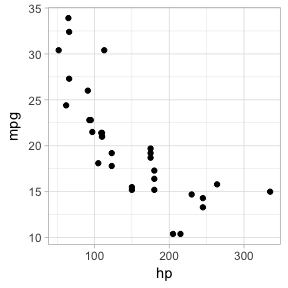## Selecting the glyph type

The “kind of graphic” is specified by the name of the graphics function. All of the `ggformula` data graphics functions have names starting with `gf_`, which is intended to remind the user that they are formula-based interfaces to `ggplot2`: `g` for `ggplot2` and `f` for “formula.” Commonly used functions include

• `gf_point()` for scatter plots
• `gf_line()` for line plots (connecting dots in a scatter plot)
• `gf_density()` or `gf_dens()` or `gf_histogram()` or `gf_freqpoly()` to display distributions of a quantitative variable
• `gf_boxplot()` or `gf_violin()` for comparing distributions side-by-side
• `gf_counts()` for bar-graph style depictions of counts.
• `gf_bar()` for more general bar-graph style graphics

The function names generally match the corresponding function name from `ggplot2`, although `gf_counts()` is a simplified special case, and `gf_dens()` is an alternative to `gf_density()` that displays the density plot slightly differently than the default in `ggplot2`.

Each of the `gf_` functions can create the coordinate axes and fill it in one operation. (In `ggplot2` nomenclature, `gf_` functions create a frame and add a geom layer, all in one operation.) This is what happens for the first `gf_` function in a chain. For subsequent `gf_` functions, new layers are added, each one “on top of” the previous layers.

## Attributes

Each of the marks in the plot is a glyph. Every glyph has graphical attributes (called aesthetics in `ggplot2`) that tell where and how to draw the glyph. In the above plot, the obvious attributes are x- and y-position:
We’ve told R to put `mpg` along the y-axis and `hp` along the x-asis, as is clear from the plot. But each point also has other attributes, including color, shape, size, stroke, fill, and alpha (transparency). We didn’t specify those in our example, so `gf_point()` uses some default values for those – in this case smallish black filled-in circles.

### Specifying attributes

In the `gf_` functions, you specify the non-position graphical attributes using an extension of the basic formula. Attributes can be set to a constant value (e.g, set the color to “blue”; set the size to 2) or they can be mapped to a variable in the data or some expression involving the variables (e.g., map the color to `sex`, so sex determines the color groupings)

Attributes are set or mapped using additional arguments.

• adding an argument of the form `attribute = value` sets `attribute` to `value`.
• adding an argument of the form `attribute = ~ expression` maps `attribute` to `expression`

where `attribute` is one of `color`, `shape`, etc., `value` is a constant (e.g. `"red"` or `0.5`, as appropriate), and `expression` may be some more general expression that can be computed using the variables in `data` (although often is is better to create a new variable in the data and to use that variable instead of an on-the-fly calculation within the plot).

The following plot, for instance,

• We use `cyl` to determine the color and `carb` to determine the size of each dot. Color and size are mapped to `cyl` and `carb`. A legend is provided to show us how the mapping is being done. (Later, we can use scales to control precisely how the mapping is done – which colors and sizes are used to represent which values of `cyl` and `carb`.)

• We also set the transparency to 50%. The gives the same value of `alpha` to all glyphs in this layer.

``gf_point(mpg ~ hp, color = ~ cyl, size = ~carb, alpha = 0.50, data = mtcars) ``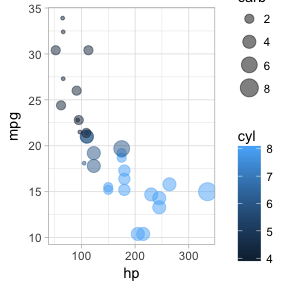Here is the same plot where mapping and setting are indicated explicitly.

``````# set alpha using a function argument instead of in the formula
gf_point(mpg ~ hp, color = ~cyl, size = ~carb, alpha = 0.50, data = mtcars) ``````### On-the-fly calculations

`ggformula` allows for on-the-fly calculations of attributes, although the default labeling of the plot is often better if we create a new variable in our data frame. In the examples below, since there are only three values for `carb`, it is easier to read the graph if we tell R to treat `cyl` as a categorical variable by converting to a factor (or to a string). Except for the labeling of the legend, these two plots are the same.

``````gf_point(mpg ~ hp,  color = ~factor(cyl), size = ~carb, alpha = 0.75, data = mtcars)
gf_point(mpg ~ hp,  color = ~cylinders, size = ~carb, alpha = 0.75,
data = mtcars %>% mutate(cylinders = factor(cyl)))``````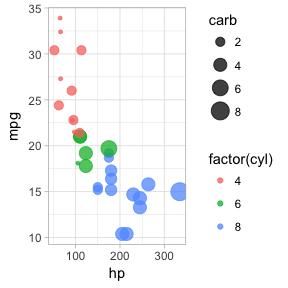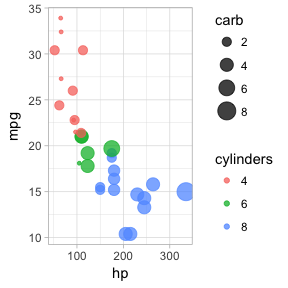## “One-variable” plots

For some plots, we only have to specify the x-position because the y-position is calculated from the x-values. Histograms, densityplots, and frequency polygons are examples. To illustrate, we’ll use density plots, but the same ideas apply to `gf_histogram()`, and `gf_freqpolygon()` as well. Note that in the one-variable density graphics, the variable whose density is to be calculated goes to the right of the tilde, in the position reserved for the x-axis variable.

``````data(Runners, package = "statisticalModeling")
Runners <- Runners %>% filter( ! is.na(net))
gf_density( ~ net, data = Runners)
gf_density( ~ net,  fill = ~sex,  alpha = 0.5, data = Runners)
# gf_dens() is similar, but there is no line at bottom/sides, and it is not "fillable"
gf_dens( ~ net, color = ~sex, alpha = 0.7, data = Runners)    ``````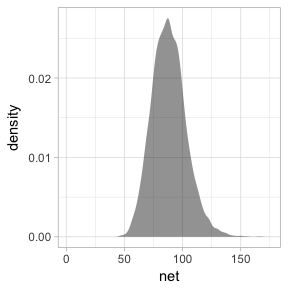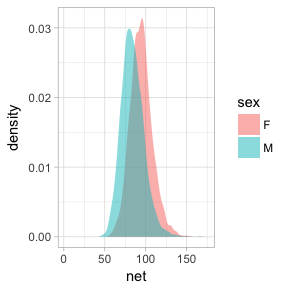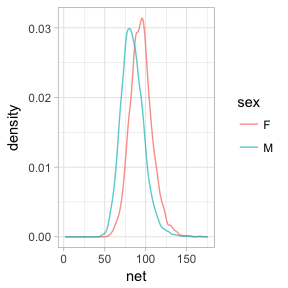Several of the plotting functions include additional arguments that do not modify attributes of individual glyphs but control some other aspect of the plot. In this case, `adjust` can be used to increase or decrease the amount of smoothing.

``````# less smoothing
gf_dens( ~ net, color = ~sex, alpha = 0.7, data = Runners, adjust = 0.25)
# more smoothing
gf_dens( ~ net, color = ~sex, alpha = 0.7, data = Runners, adjust = 4)     ``````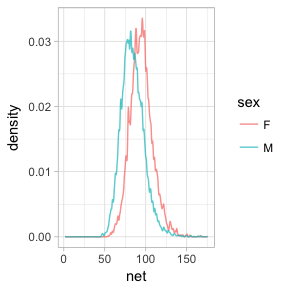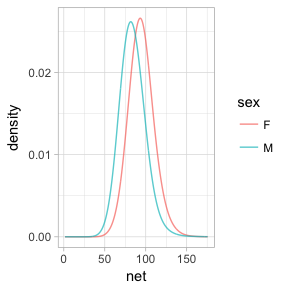## Position

When the `fill` or `color` or `group` aesthetics are mapped to a variable, the default behavior is to lay the group-wise densities on top of one another. Other behavior is also available by using `position` in the formula. Using the value `"stack"` causes the densities to be laid one on top of another, so that the overall height of the stack is the density across all groups. The value `"fill"` produces a conditional probability graphic.

``````gf_density( ~ net, fill = ~sex, color = NA, position = "stack", data = Runners)
gf_density( ~ net, fill = ~sex, color = NA, position = "fill", data = Runners, adjust = 2)``````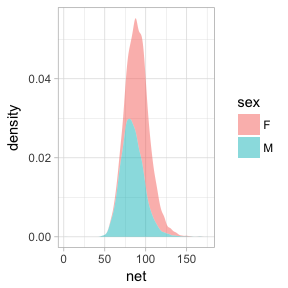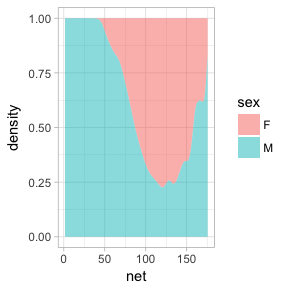Similar commands can be constructed with `gf_histogram()` and `gf_freqpoly()`, but note that `color`, not `fill`, is the active attribute for frequency polygons. It’s also rarely good to overlay histograms on top of one another – better to use a density plot or a frequency polygon for that application.

## More 2-variable plots

### Using jitter and transparency to handle overlapping cases

Sometimes you have so many points in a scatter plot that they obscure one another. The `ggplot2` system provides two easy ways to deal with this: translucency and jittering.

Use `alpha = 0.5` to make the points semi-translucent. If there are many points overlapping at one point, a much smaller value of alpha, say `alpha = 0.01`. We’ve already seen this above.

Using `gf_jitter()` in place of `gf_point()` will move the plotted points to reduce overlap. Jitter and transparency can be used together as well.

``````gf_point(age ~ sex, alpha = 0.05, data = Runners)
gf_jitter(age ~ sex, alpha = 0.05, data = Runners)``````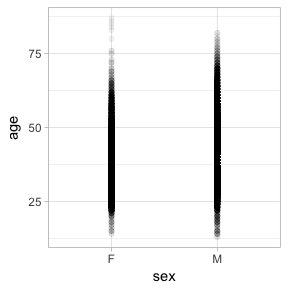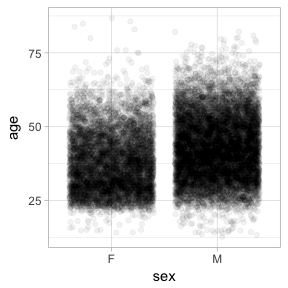### Box and Whisker plots

Box and whisker plots show the distribution of a quantitative variable as a function of a categorical variable. The formula used in `gf_boxplot()` should have the quantitative variable to the left of the tilde. (To make horizontal boxplots using `ggplot2` you have to make vertical boxplots and then flip the coordinates with `coord_flip()`.)

``````gf_boxplot(net ~ sex, color = "red", data = Runners)
gf_boxplot(net ~ sex, color = ~start_position, data = Runners)``````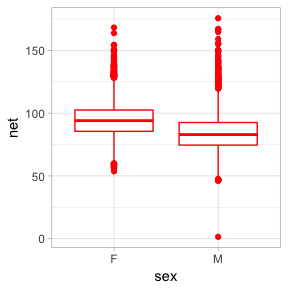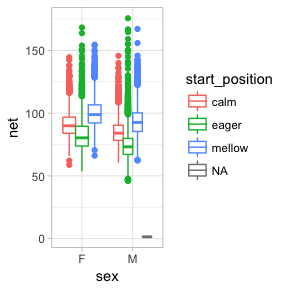This plot may surprise you.

``gf_boxplot(net ~ year, data = Runners)``
``## Warning: Continuous x aesthetic -- did you forget aes(group=...)?``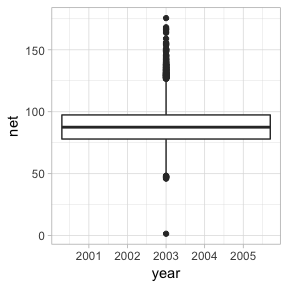This plot is placing a single box and whisker plot at the mean value of `year`. The warning message suggests that we need to tell R how to form the groups when using a quantitative variable for `x`. It suggests using the `group` aesthetic, and sometimes, this is just what we want.

``gf_boxplot(net ~ year, group = ~ year, data = Runners)``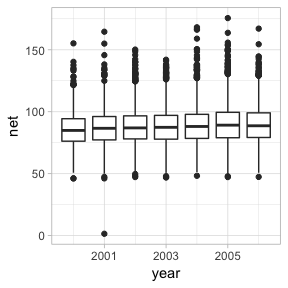But often, is is better to convert a discrete quantitative variable used for grouping into a categorical variable (a factor or character vector). This can be done in several ways:

``````# add a new variable to the data
Runners\$the_year <- as.character(Runners\$year)               # in base R
Runners <- Runners %>% mutate(the_year = as.character(year)) # in dplyr
gf_boxplot(net ~ the_year, color = ~sex, data = Runners)
# or do it on the fly
gf_boxplot(net ~ factor(year), color = ~sex, data = Runners)``````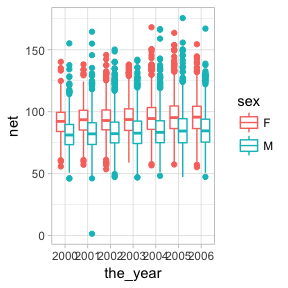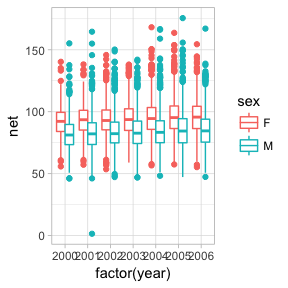### 2-dimensional density plots

Two-dimensional plots of density also have both a left and right component to the formula.

``````gf_density_2d(net ~ age, data = Runners)
gf_hex(net ~ age, data = Runners)``````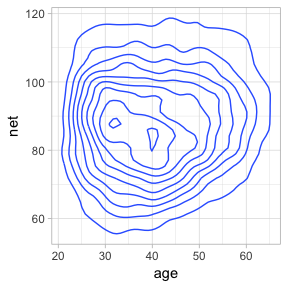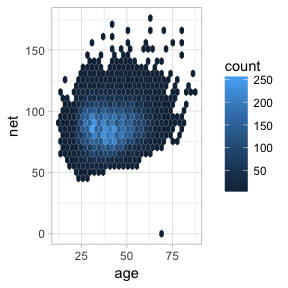### Paths and lines

The `ggplot2` system offers two ways to connect points. `gf_line()` ignores the order of the points in the data, and draws the line going from left to right. `gf_path()` goes from point to point according to the order in the data. Both forms can use a `color` or `group` aesthetic as a flag to draw groupwise lines.

``````# create a categorical variable
mtcars <- mtcars %>% mutate(n_cylinders = as.character(cyl))
gf_line(mpg ~ hp, data = mtcars)
gf_path(mpg ~ hp, data = mtcars)
gf_line(mpg ~ hp, color = ~n_cylinders, data = mtcars)``````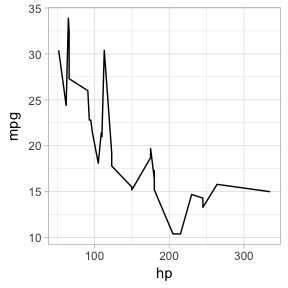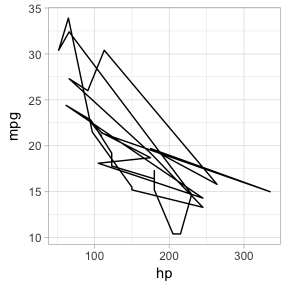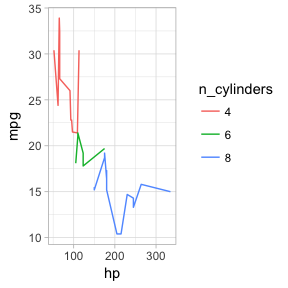The above are examples of bad plots. The viewer is unnecessarily distracted by the zigs and zags in the connecting lines. It would be better to use `gf_point()` here, but then you wouldn’t see how `gf_line()` and `gf_path()` work!

Here’s a more useful example. We begin with a scatter plot showing the number of live births in the US for each day of 1978.

``````library(mosaicData)
gf_point(births ~ date, data = Births78)``````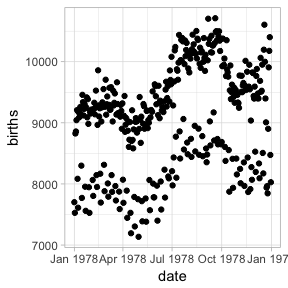Can this interesting pattern be explained by a weekday/weekend effect? We could use color to show the days of the week.

``````library(mosaicData)
gf_point(births ~ date, color = ~wday, data = Births78)``````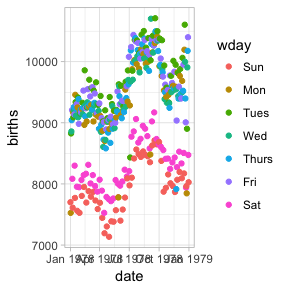Converting to a line plot highlights the pattern and makes it easier to spot the unusual days.

``gf_line(births ~ date, color = ~wday, data = Births78)``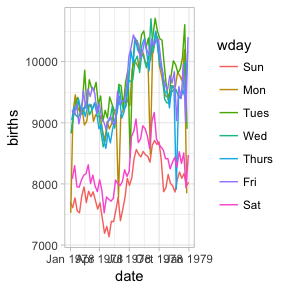## Plots with more than 2 positional attributes

Some layers require more than two attributes. Typically this happens when the glyphs of a layer are complex objects that could have been made using multiple layers, but belong together conceptually. Examples include

• `gf_pointrange()` – plots a dot flanked by a line segment.
• `gf_linrange()` – like `gf_pointrange()` but without the point.
• `gf_errorbar()` – vertical error bars.
• `gf_errorbarh()` – horizontal error bars.
• `gf_ribbon()` – a band between a line above and line below.

Often these are used to depict some sort of estimate of uncertainty in a measurement or a prediction, but they can be used to represent any data of the correct form. Here we will use `gf_linerange()` and `gf_ribbon()` to indicate the high and low temperatures in New York for the first few months of 2013.

``require(weatherData)``
``## Loading required package: weatherData``
``````Temps <- NewYork2013 %>%
mutate(date = lubridate::date(Time),
month = lubridate::month(Time)) %>%
filter(month <= 4) %>%
group_by(date) %>%
summarise(
hi = max(Temperature, na.rm = TRUE),
lo = min(Temperature, na.rm = TRUE)
)
gf_linerange(lo + hi  ~ date, color = ~hi, data = Temps)
gf_ribbon(lo + hi ~ date, data = Temps, color = "navy", alpha = 0.3)``````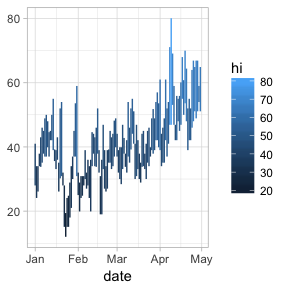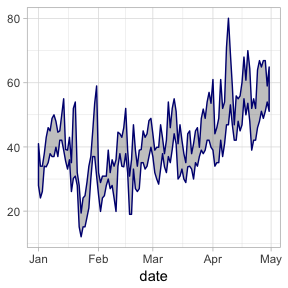## Positions and Stats

### Positions

`position_dodge()`, `position_jitter()`, and `position_jitterdodge()` can be used to adjust the positions at which glyphs are placed. Jittering adds some random noise and can be useful when many observations have the same value. Dodging moves groups of glyphs a fixed difference to make it easier to distinguish the groups.

``````gf_point(length ~ sex, color = ~ domhand, data = KidsFeet,
position = position_jitterdodge(jitter.width = 0.2, dodge.width = 0.4))``````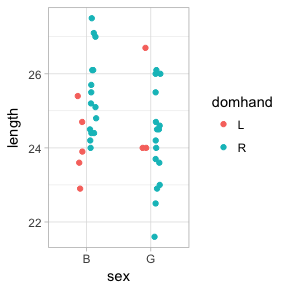### Stats

A stat is a transformation that is applied to the data before a plot is generated. Several of the plots we have seen have made use of stats.

• `gf_histogram()` uses `stat_bin()` to bin the data and count the number of observations in each bin. It is equivalent to
``gf_bar( ~ age, data = HELPrct, stat = "bin")``
``## `stat_bin()` using `bins = 30`. Pick better value with `binwidth`.``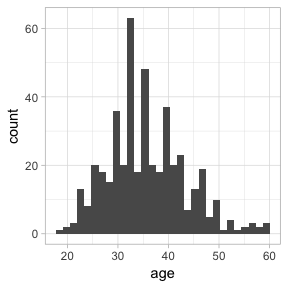* `gf_boxplot()` uses `stat_boxplot()` to compute the five-number summary on which the boxplot is based.

• `gf_violin()`, `gf_density()`, and `gf_density2d()` use stats to compute a sequence of points along an estimated density. It is these points, and the not the raw data that are used to create the plot.

Mostly, the stats selected by default are just the ones you need. But sometimes it is useful to select a different stat. The `stat_summary()` and `stat_summary_bin()` stats are particularly useful in this respect. These stats use a function to aggregate over unique values of `x` or over bins of `x` values and save the user needing to do that data transformation manually.

The default function applied in each group is `mean_se()`, which computes the mean (and the mean plus and minus one standard error) This makes it simple to create an “interaction plot”.

``````gf_jitter(length ~ sex, color = ~domhand, data = KidsFeet,
width = 0.1, height = 0) %>%
gf_line(length ~ sex, color = ~domhand, data = KidsFeet,
group = ~domhand, stat="summary")``````
``## No summary function supplied, defaulting to `mean_se()``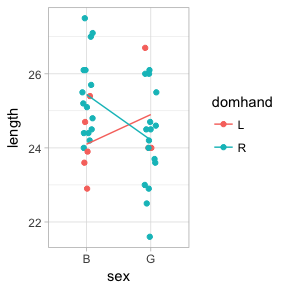The other two values computed by `mean_se()` are available as `..ymin..` and `..ymax..`.

``````gf_jitter(length ~ sex, color = ~domhand, data = KidsFeet,
width = 0.1, height = 0, alpha = 0.3) %>%
gf_pointrange(length + ..ymin.. + ..ymax.. ~ sex,
color = ~domhand, data = KidsFeet,
group = ~domhand, stat="summary")``````
``## No summary function supplied, defaulting to `mean_se()``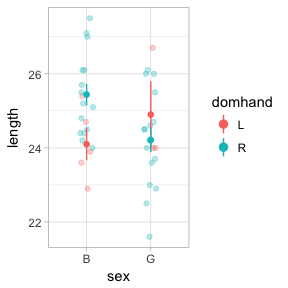Custom functions can be used by defining `fun.y`, `fun.ymin`, and `fun.ymax`, or a single function `fun.data` that returns a data frame with variables named `y`, `ymin`, and `ymax`.

``````gf_point(length ~ sex, color = ~domhand, data = KidsFeet,
width = 0.1, height = 0, alpha = 0.5,
position = position_jitterdodge(jitter.width = 0.2, dodge.width = 0.3)) %>%
gf_pointrange(length + ..ymin.. + ..ymax.. ~ sex,
color = ~domhand, data = KidsFeet,
group = ~domhand, stat="summary",
fun.y = median, fun.ymin = min, fun.ymax = max,
position = position_dodge(width = 0.6))``````